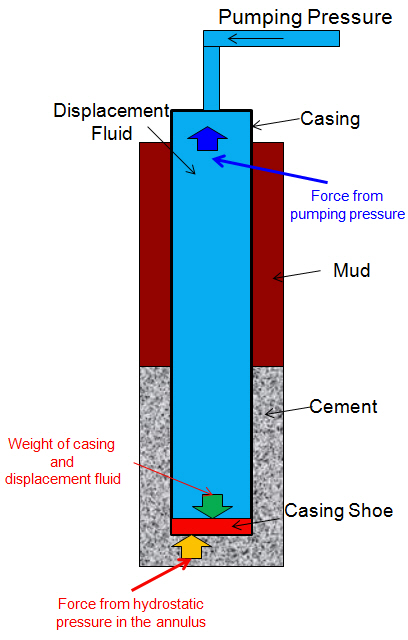# Hydraulicing Casing Calculation Example

For the previous topic, Hydraulicing Casing (pressure to lift the casing while cementing), you already know the concept. This topic will demonstrate you how to figure out if the casing will be hydraulically lifted while pumping cement.

Example:

Casing 9-5/8”, 40 ppf (pound per foot), ID of casing = 8.835”

Casing is set at 3,200’MD/3,000’TVD

Top of cement at 600’MD/550’TVD

Previous casing shoe (13-3/8”) = 1000’MD/900TVD

Cement weight = 14.0 ppg

Mud weight = 9.5 ppg

Displacement fluid weight (Brine) = 8.4 ppg

What is the condition at the static condition after cement in place?

What is the maximum pressure that we can apply before the casing is hydraulically pumped out of the well?

## Under a static conditionForces downward are Weight of casing and Wdf + Weight of displacement fluid.

Force upward is  force due to hydrostatic pressure in the annulus.

The equation is showed below:

∆F = (Wc+Wdf) – (HPann x A)

Where:

Wc = Weight of casing

Wdf = Weight of displacement fluid

HPann = Hydrostatic Pressure in the annulus

A = Cross section area of casing

We can put the numbers into these equations like this.

Wc = 40 x 3200 = 128,000 lb

Wdf = Hydrostatic pressure x Internal area of casing

Wdf = (0.052 x 8.4 x 3000) x (π÷4 x (8.8352) = 80,335 lb

HPann = (0.052 x 9.5 x 550) + (0.052 x 14 x (3000-550)) = 2,055 psi

HPann x A = 2,055 x (π÷4 x (9.6252) = 149,521 lb

∆F = (128,000 + 80,335) – 149,521 = 58,814 lb

There is NO problem under static condition because downward force is more that upward force.

## Under a dynamic condition

While pumping, the hydraulic pressure will force upward therefore the equation will be like thisWe still use the same concept but this time we must add a force term generated by pumping pressure (Ppump x a). This is the equation.

∆F = (Wc+Wdf) – (HPann x A + Ppump x a)

Where:

Wc = Weight of casing

Wdf = Weight of displacement fluid

HPann = Hydrostatic Pressure in the annulus

a = Cross section area of inside of casing

Ppump = pumping pressure

A = cross sectiona area of casing

We can determine the maximum pumping pressure before the casing will be lifted by substituting ∆F to 0.

When ∆F = 0, the casing is about to be hydraulically lifted out of the wellbore so you can write the new equation in new term like this:

0 = (Wc+Wdf) – (HPann x A + Ppump x a)

Ppump x a = (Wc+Wdf) – (HPann x A )

Ppump = [(Wc+Wdf) – (HPann x A )] ÷ a

Ppump from this relationship is maximum pumping pressure.

From the previous calculation, you already have the following figure:

Wc = 128,000 lb

Wdf = 80,335 lb

HPann x A = 149,521 lb

Ppump x (π÷4 x (8.835²)) = (128,000 + 80,335) – 149,521

Ppump = 959 psi

## Conclusion

What is the condition at the static condition after cement in place?
No movement

What is the maximum pressure that we can apply before the casing is hydraulically pumped out of the well?

The maximum pressure that you can have while pumping is 959 psi.

I wish you can apply this calculation to your operation.

Ref books:Cementing Technology Books

Share the joyWorking in the oil field and loving to share knowledge.

### 3 Responses to Hydraulicing Casing Calculation Example

1.Dane says:

Awesome, thanks !

2.Condet says:

Thanks.. Very useful calculation, but is there any effect bouyancy to weight of casing..?

•DrillingFormulas.Com says: